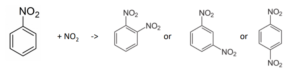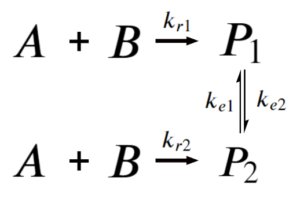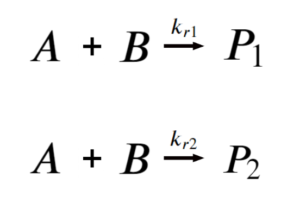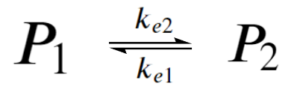# 19 Kinetic & Thermodynamic Control

Learning Objectives

By the end of this section, you should be able to:

Understand kinetic and thermodynamic control

Reactants can sometimes give rise to a variety of products.

Consider the nitration of nitrobenzene:The relative portion of the products before reaching equilibrium is given by the ratio of the rates of production.

$A+B→P_{1}$ where $r_{P1}=k_{r1}[A][B]$

$A+B→P_{2}$ where $r_{P2}=k_{r2}[A][B]$

Here, before equilibrium:

 $\frac{[P_{2}]}{[P_{1}]}=\frac{k_{r,2}}{k_{r,1}}$

This is called kinetic control, and it is dictated by reaction rates.
As opposed to thermodynamic control, which is dictated by reaction equilibrium (after a long time):

Say we have the system:If $k_{e1},k_{e2}\text{<<}k_{r1},k_{r2}$

Then at any time before the equilibrium reaction start severely affecting product concentration, the reaction simplifies to:$\frac{[P_{1}]}{[P_{2}]}=\frac{k_{r1}}{k_{r2}}$

The reaction is kinetically controlled: the amount of products depends on the rates of reaction.

Proof to show: $\frac{[P_{1}]}{[P_{2}]}=\frac{k_{r1}}{k_{r2}}$
\begin{align*}
r_{P1}=k_{r1}[A][B]&=\frac{d[P1]}{dt}\\
r_{P2}=k_{r2}[A][B]&=\frac{d[P2]}{dt}
\end{align*}

Say that both $P_{1}$, $P_{2}$ start at a concentration of 0. We can express the change in concentration for $P_{1}$ and $P_{2}$ at any time before [A][B] reaches 0. Note that once [A][B] reaches 0, the equilibrium reaction starts to dominate as we no longer have forward reactions that consume A and B to produce P1 and P2.

$\frac{[P1]}{[P2]}=\frac{\frac{d[P_{1}]}{dt}}{\frac{d[P_{2}]}{dt}}=\frac{k_{r1}[A][B]}{k_{r2}[A][B]}=\frac{k_{r1}}{k_{r2}}$

If $k_{e1},k_{e2}>>k_{r1},k_{r2}$

Then at any given time, this reaction simplifies to:$\frac{[P_{1}]}{[P_{2}]}=\frac{k_{e1}}{k_{e2}}$

The reaction is thermodynamically controlled : the amount of products depends on the equilibrium state.

Proof to show: $\frac{[P_{1}]}{[P_{2}]}=\frac{k_{e1}}{k_{e2}}$:
At equilibrium:

Forward reaction rate: $\frac{d[P2]}{dt}=k_{e2}[P1]$

Reverse reaction rate: $\frac{d[P1]}{dt}=k_{e1}[P2]$

At equilibrium, the forward and reverse reaction rates are equal:

\begin{align*}
k_{e2}[P1] & = k_{e1}[P2]\\
\frac{[P_{1}]}{[P_{2}]} & = \frac{k_{e1}}{k_{e2}}
\end{align*}